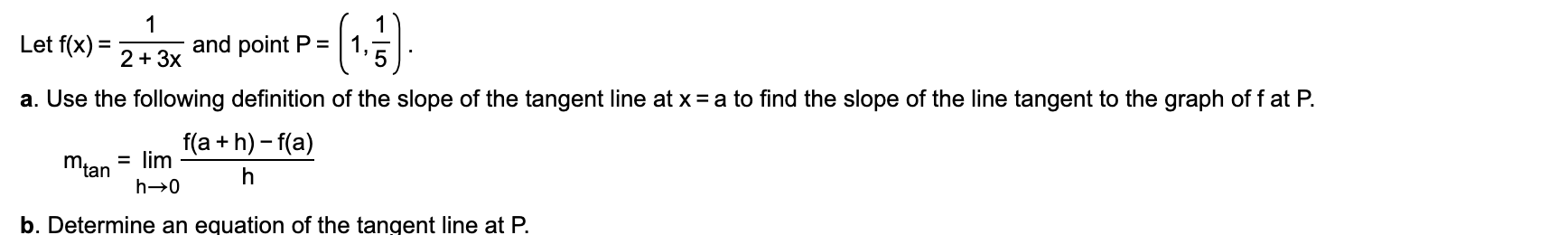# Let f(x) =and point P =%D2 + 3xa. Use the following definition of the slope of the tangent line at x = a to find the slope of the line tangent to the graph of f at P.f(a + h) - f(a)= limMianb. Determine an equation of the tangent line at P.-|5

Question
12 viewshelp_outlineImage TranscriptioncloseLet f(x) = and point P = %D 2 + 3x a. Use the following definition of the slope of the tangent line at x = a to find the slope of the line tangent to the graph of f at P. f(a + h) - f(a) = lim Mian b. Determine an equation of the tangent line at P. -|5 fullscreen
check_circle

Step 1

Given:

Step 2

Calculation:

Step 3

Find the slope of t...

### Want to see the full answer?

See Solution

#### Want to see this answer and more?

Solutions are written by subject experts who are available 24/7. Questions are typically answered within 1 hour.*

See Solution
*Response times may vary by subject and question.
Tagged in

### Other